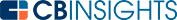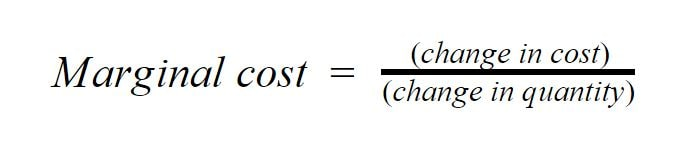# How to Calculate Marginal CostWhenever a business increases the production level of a product, there is an associated change in the production cost. Marginal cost takes this into account. Marginal cost is an important measure for determining whether a company has reached its optimum production level.

Essentially, a lower marginal cost means that the producer could potentially gain more profit.

## Marginal cost calculation

When calculating the marginal cost of increasing the production of an item, a business needs to take into consideration the following:

• Change in the cost associated with item production: When the production level of an item changes, production costs either increase or decrease. This could happen, for example, if more workers are needed at a time when the production of an item is increased. This would increase the production cost since this would cause a wage increase. The change is determined by simply subtracting the cost of the first output run from the cost of the new output run.
• Change in the quantity of the item being produced: When the production level changes, the amount of the item being produced either increases or decreases. The change in quantity is determined by simply subtracting the number of items produced during the initial run from the number of goods produced after the production level changes.

With this in mind, you can use the formula to calculate the marginal cost associated with changing production levels:## Marginal cost example

A computer technology company called Frontier Technologies usually produces 20,000 units annually. This results in a production bill of \$1M. This year, due to market demand increasing, Frontier needed to produce more units. This resulted in increased production costs to purchase additional components and hire new workers.

Overall, the number of units produced increased to 30,000 while costs increased to \$2.5M. In order for Frontier’s analysts to calculate the marginal cost as a result of this increased production, the formula above is used.

First, the analysts note the following information:

• Change in cost: The change in cost is \$2.5M - \$1M = \$1.5M.
• Change in quantity: The change in quantity is 30,000 - 20,000 = 10,000 units.

With this information at hand, the marginal cost can then be calculated.This essentially means that it costs an additional \$150 per unit to produce the extra 10,000 units.

## Importance of marginal cost for businesses

Marginal cost lets businesses know if increasing an item’s production is justified. If the price an item is sold for is higher than the marginal cost of an item, then a business stands to see increased profits. However, if the selling price is lower than the marginal cost, then losses will occur.

Marginal cost can also help businesses to see if their production level is optimized. To grasp this better, we need to talk about marginal revenue. This accounts for the change in revenue when production levels change. The formula for marginal revenue is as follows:If the marginal revenue as a result of a production change equals the marginal cost, then a business’ production is optimized and it stands to make more profits. For this reason, both marginal cost and marginal revenue are important metrics for businesses and their analysts to monitor.

Calculating marginal cost allows businesses to calculate the cost per unit for every additional unit when a company increases production of an item. This helps them to gauge if the change will increase or decrease their profits.

You may also like# Corporate InnovationCorporate StrategyCorporate Venture CapitalVenture CapitalLove Notes